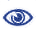# The Prague Stringology Conference 2009

Searching for Jumbled Patterns in Strings

 Abstract: The Parikh vector of a string s over a finite ordered alphabet Σ = a1,...,aσ is defined as the vector of multiplicities of the characters, i.e. p(s) = (p1,...,pσ), where pi = |j | sj = ai|. Parikh vector q occurs in s if s has a substring t with p(t) = q. The problem of searching for a query q in a text s of length n can be solved simply and optimally with a sliding window approach in O(n) time. We present two new algorithms for the case where the text is fixed and many queries arrive over time. The first algorithm finds all occurrences of a given Parikh vector in a text (over a fixed alphabet of size σ ≥  2) and appears to have a sub-linear expected time complexity. The second algorithm only decides whether a given Parikh vector appears in a binary text; it iteratively constructs a linear size data structure which then allows answering queries in constant time, for many queries even during the construction phase.

 Download paper:PostScript PDF BibTeX reference
 Download presentation: